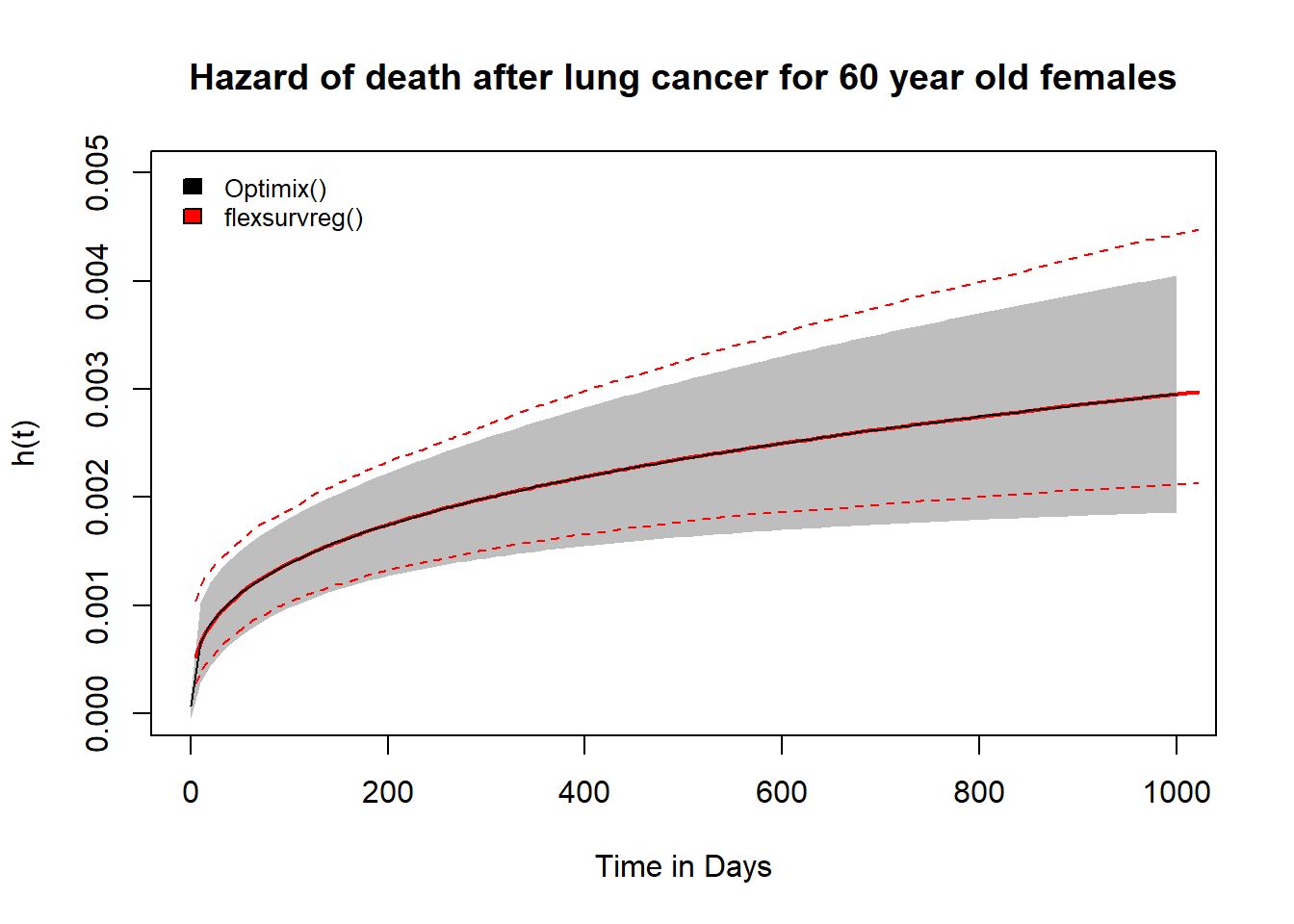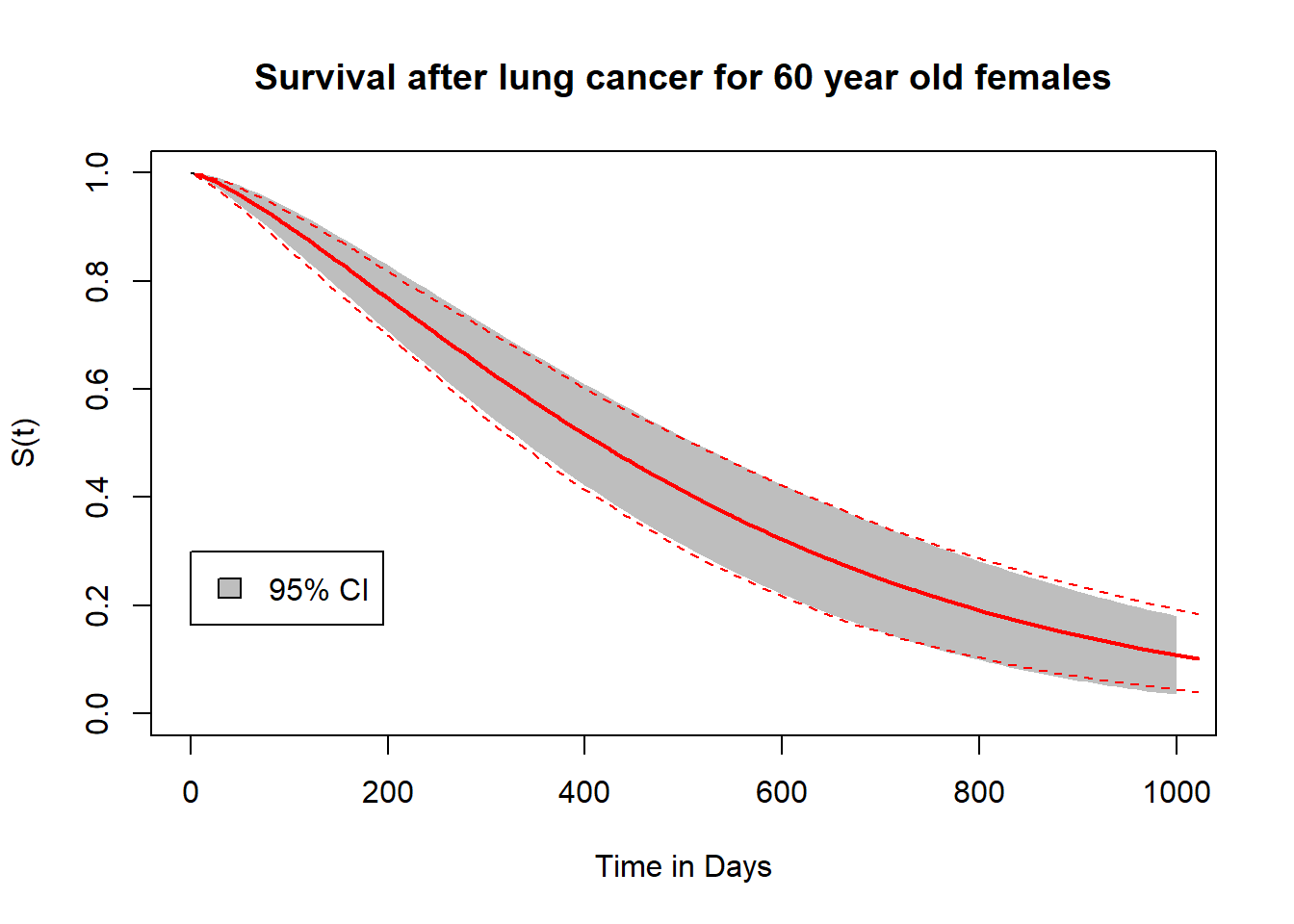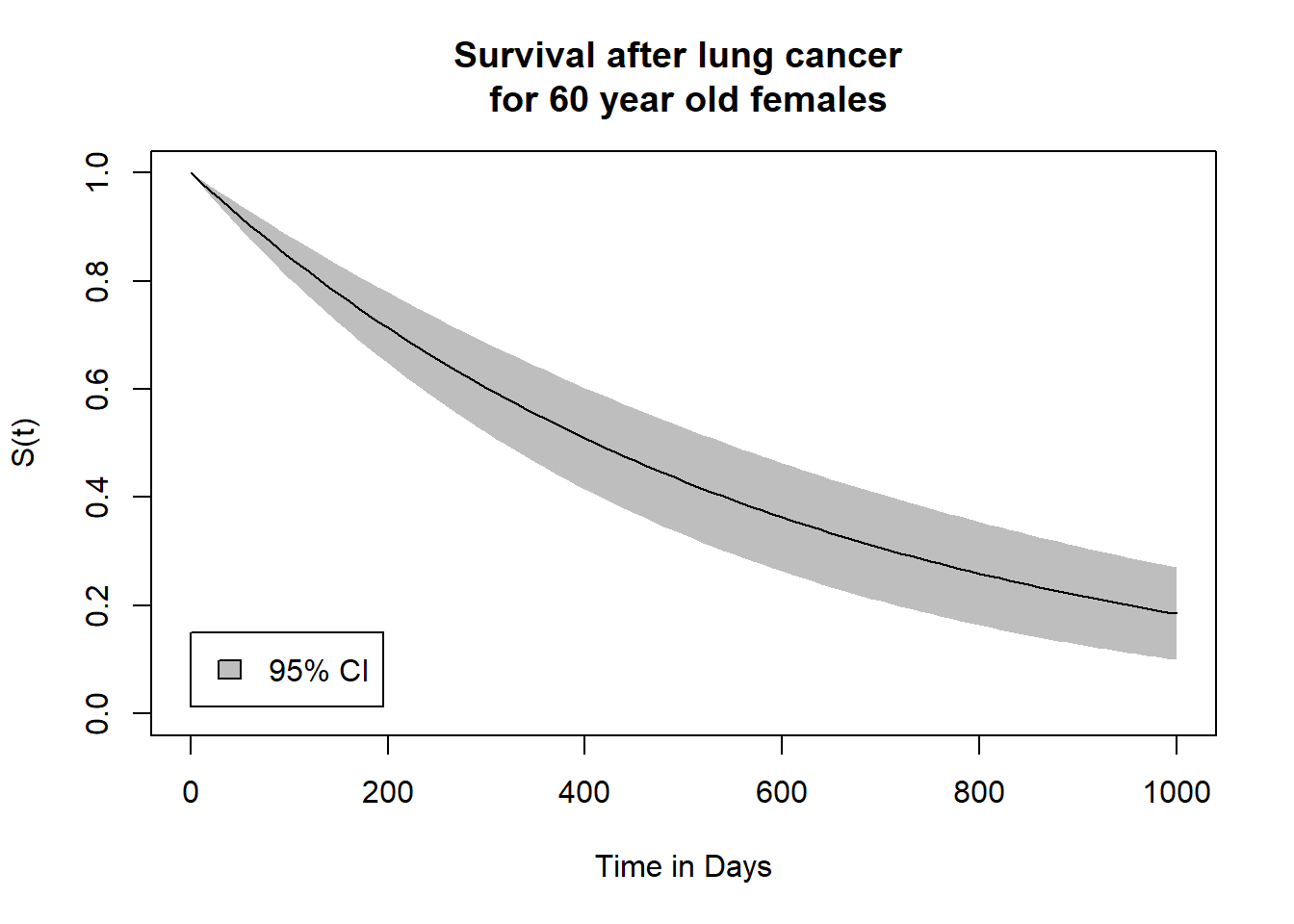# Articles by R | Joshua Entrop

### {ExclusionTable} a package for keeping track of exclusions and inclusions

October 31, 2021 |

In today’s blog post we will take a look at a package that allows you to keep track of the number of observations that you in- or exclude from your dataset or study population. In epidemiological studies we often want to limit our study population based on some inclusion ... [Read more...]

### Optimisation of a stratified Cox model using Optimx()

April 11, 2021 |

In this blog post we are going to fit a stratified Cox regression model by optimising its likelihood function with Optimx::optimx(). Stratified Cox regression models allow one to relax the assumption of proportional hazards over time between differen... [Read more...]

### Optimisation of a Cox proportional hazard model using Optimx()

February 27, 2021 |

In this blog post we will optimise a Cox proportional hazard model using a maximum likelihood estimation (MLE) method. For this we are first going to define the likelihood function of our Cox model and its partial first derivatives, sometimes called ... [Read more...]

### Comparing the confidence intervals of a Weibull model estimated with flexsurvreg() and optimx()

October 24, 2020 |

This blog post is a follow up on my previous post on optimising a Weibull regression model using optimx(). This time I’ll try to find a solution for the discrepancy between the confidence interval estimates of the Weibull hazard function estimated wit...### Optimisation of a Weibull survival model using Optimx() in R

September 27, 2020 |

In this blog post we will optimise a Weibull regression model by maximising its likelihood function using optimx() from the {optimx} package in R. In my previous blog post I showed how to optimise a Poisson regression model in the same manner. Optimis...### Optimisation of a Poisson survival model using Optimx in R

July 21, 2020 |

In this blog post, we will fit a Poisson regression model by maximising its likelihood function using optimx() in R. As an example we will use the lung cancer data set included in the {survival} package. The data set includes information on 228 lung cancer patients from the North Central Cancer ...### Estimating Standard Errors for a Logistic Regression Model optimised with Optimx in R

June 24, 2020 |

In my last post I estimated the point estimates for a logistic regression model using optimx() from the optimx package in R. In this post I would like to contine with this model an try to find the standard error (SE) for the derived estimates. Uncertainty is probably the most ... [Read more...]

### Optimisation of a Logistic Regression Model using Optimx in R

May 26, 2020 |

In my last post I used the optim() command to optimise a linear regression model. In this post, I am going to take that approach a little further and optimise a logistic regression model in the same manner. Thanks to John C. Nash, I got a first glimpse into the ... [Read more...]

### Optimisation of a Linear Regression Model in R

April 25, 2020 |

In this post I would like to show how to manually optimise a linear regression model using the optim() command in R. Usually if you learn how to fit a linear regression model in R, you would learn how to use the lm() command to do this. However, if you ... [Read more...]